# QEasingCurve#

The `QEasingCurve` class provides easing curves for controlling animation. More

New in version 4.6.

## Synopsis#

### Functions#

Note

This documentation may contain snippets that were automatically translated from C++ to Python. We always welcome contributions to the snippet translation. If you see an issue with the translation, you can also let us know by creating a ticket on https:/bugreports.qt.io/projects/PYSIDE

## Detailed Description#

Warning

This section contains snippets that were automatically translated from C++ to Python and may contain errors.

Easing curves describe a function that controls how the speed of the interpolation between 0 and 1 should be. Easing curves allow transitions from one value to another to appear more natural than a simple constant speed would allow. The `QEasingCurve` class is usually used in conjunction with the `QVariantAnimation` and `QPropertyAnimation` classes but can be used on its own. It is usually used to accelerate the interpolation from zero velocity (ease in) or decelerate to zero velocity (ease out). Ease in and ease out can also be combined in the same easing curve.

To calculate the speed of the interpolation, the easing curve provides the function `valueForProgress()` , where the `progress` argument specifies the progress of the interpolation: 0 is the start value of the interpolation, 1 is the end value of the interpolation. The returned value is the effective progress of the interpolation. If the returned value is the same as the input value for all input values the easing curve is a linear curve. This is the default behaviour.

For example,

```easing = QEasingCurve(QEasingCurve.InOutQuad)
for t in range(0.0, 1.0):
qWarning() << "Effective progress" << t << "is"
<< easing.valueForProgress(t)
```

will print the effective progress of the interpolation between 0 and 1.

When using a `QPropertyAnimation` , the associated easing curve will be used to control the progress of the interpolation between startValue and endValue:

```animation = QPropertyAnimation()
animation.setStartValue(0)
animation.setEndValue(1000)
animation.setDuration(1000)
```

The ability to set an amplitude, overshoot, or period depends on the `QEasingCurve` type. Amplitude access is available to curves that behave as springs such as elastic and bounce curves. Changing the amplitude changes the height of the curve. Period access is only available to elastic curves and setting a higher period slows the rate of bounce. Only curves that have “boomerang” behaviors such as the `InBack` , `OutBack` , `InOutBack` , and `OutInBack` have overshoot settings. These curves will interpolate beyond the end points and return to the end point, acting similar to a boomerang.

The Easing Curves Example contains samples of `QEasingCurve` types and lets you change the curve settings.

class PySide6.QtCore.QEasingCurve([type=QEasingCurve.Type.Linear])#

PySide6.QtCore.QEasingCurve(other)

Parameters:

Constructs an easing curve of the given `type`.

Construct a copy of `other`.

PySide6.QtCore.QEasingCurve.Type#

The type of easing curve.

Constant

Description

QEasingCurve.Linear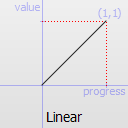Easing curve for a linear (t) function: velocity is constant.

Constant

Description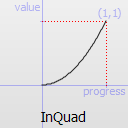Easing curve for a quadratic (t^2) function: accelerating from zero velocity.

Constant

Description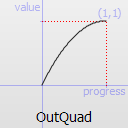Easing curve for a quadratic (t^2) function: decelerating to zero velocity.

Constant

Description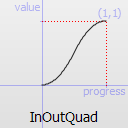Easing curve for a quadratic (t^2) function: acceleration until halfway, then deceleration.

Constant

Description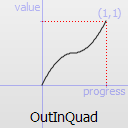Easing curve for a quadratic (t^2) function: deceleration until halfway, then acceleration.

Constant

Description

QEasingCurve.InCubic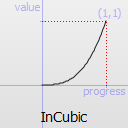Easing curve for a cubic (t^3) function: accelerating from zero velocity.

Constant

Description

QEasingCurve.OutCubic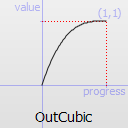Easing curve for a cubic (t^3) function: decelerating to zero velocity.

Constant

Description

QEasingCurve.InOutCubic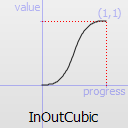Easing curve for a cubic (t^3) function: acceleration until halfway, then deceleration.

Constant

Description

QEasingCurve.OutInCubic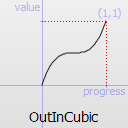Easing curve for a cubic (t^3) function: deceleration until halfway, then acceleration.

Constant

Description

QEasingCurve.InQuart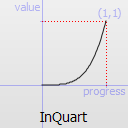Easing curve for a quartic (t^4) function: accelerating from zero velocity.

Constant

Description

QEasingCurve.OutQuart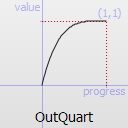Easing curve for a quartic (t^4) function: decelerating to zero velocity.

Constant

Description

QEasingCurve.InOutQuart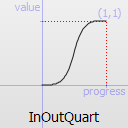Easing curve for a quartic (t^4) function: acceleration until halfway, then deceleration.

Constant

Description

QEasingCurve.OutInQuart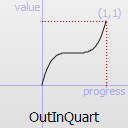Easing curve for a quartic (t^4) function: deceleration until halfway, then acceleration.

Constant

Description

QEasingCurve.InQuint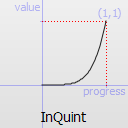Easing curve for a quintic (t^5) easing in: accelerating from zero velocity.

Constant

Description

QEasingCurve.OutQuint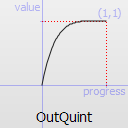Easing curve for a quintic (t^5) function: decelerating to zero velocity.

Constant

Description

QEasingCurve.InOutQuint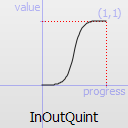Easing curve for a quintic (t^5) function: acceleration until halfway, then deceleration.

Constant

Description

QEasingCurve.OutInQuint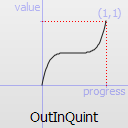Easing curve for a quintic (t^5) function: deceleration until halfway, then acceleration.

Constant

Description

QEasingCurve.InSine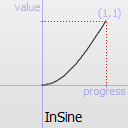Easing curve for a sinusoidal (sin(t)) function: accelerating from zero velocity.

Constant

Description

QEasingCurve.OutSine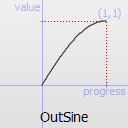Easing curve for a sinusoidal (sin(t)) function: decelerating to zero velocity.

Constant

Description

QEasingCurve.InOutSine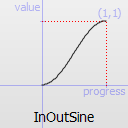Easing curve for a sinusoidal (sin(t)) function: acceleration until halfway, then deceleration.

Constant

Description

QEasingCurve.OutInSine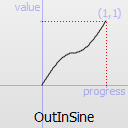Easing curve for a sinusoidal (sin(t)) function: deceleration until halfway, then acceleration.

Constant

Description

QEasingCurve.InExpo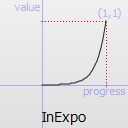Easing curve for an exponential (2^t) function: accelerating from zero velocity.

Constant

Description

QEasingCurve.OutExpo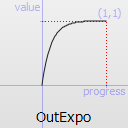Easing curve for an exponential (2^t) function: decelerating to zero velocity.

Constant

Description

QEasingCurve.InOutExpo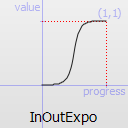Easing curve for an exponential (2^t) function: acceleration until halfway, then deceleration.

Constant

Description

QEasingCurve.OutInExpo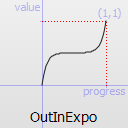Easing curve for an exponential (2^t) function: deceleration until halfway, then acceleration.

Constant

Description

QEasingCurve.InCirc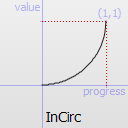Easing curve for a circular (sqrt(1-t^2)) function: accelerating from zero velocity.

Constant

Description

QEasingCurve.OutCirc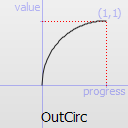Easing curve for a circular (sqrt(1-t^2)) function: decelerating to zero velocity.

Constant

Description

QEasingCurve.InOutCirc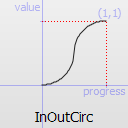Easing curve for a circular (sqrt(1-t^2)) function: acceleration until halfway, then deceleration.

Constant

Description

QEasingCurve.OutInCirc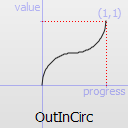Easing curve for a circular (sqrt(1-t^2)) function: deceleration until halfway, then acceleration.

Constant

Description

QEasingCurve.InElastic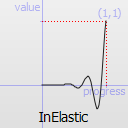Easing curve for an elastic (exponentially decaying sine wave) function: accelerating from zero velocity. The peak amplitude can be set with the amplitude parameter, and the period of decay by the period parameter.

Constant

Description

QEasingCurve.OutElastic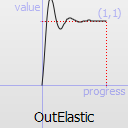Easing curve for an elastic (exponentially decaying sine wave) function: decelerating to zero velocity. The peak amplitude can be set with the amplitude parameter, and the period of decay by the period parameter.

Constant

Description

QEasingCurve.InOutElastic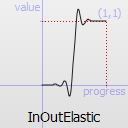Easing curve for an elastic (exponentially decaying sine wave) function: acceleration until halfway, then deceleration.

Constant

Description

QEasingCurve.OutInElastic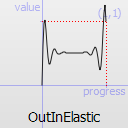Easing curve for an elastic (exponentially decaying sine wave) function: deceleration until halfway, then acceleration.

Constant

Description

QEasingCurve.InBack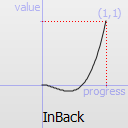Easing curve for a back (overshooting cubic function: (s+1)*t^3 - s*t^2) easing in: accelerating from zero velocity.

Constant

Description

QEasingCurve.OutBack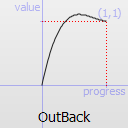Easing curve for a back (overshooting cubic function: (s+1)*t^3 - s*t^2) easing out: decelerating to zero velocity.

Constant

Description

QEasingCurve.InOutBack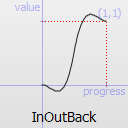Easing curve for a back (overshooting cubic function: (s+1)*t^3 - s*t^2) easing in/out: acceleration until halfway, then deceleration.

Constant

Description

QEasingCurve.OutInBack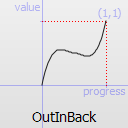Easing curve for a back (overshooting cubic easing: (s+1)*t^3 - s*t^2) easing out/in: deceleration until halfway, then acceleration.

Constant

Description

QEasingCurve.InBounce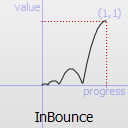Easing curve for a bounce (exponentially decaying parabolic bounce) function: accelerating from zero velocity.

Constant

Description

QEasingCurve.OutBounce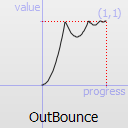Easing curve for a bounce (exponentially decaying parabolic bounce) function: decelerating from zero velocity.

Constant

Description

QEasingCurve.InOutBounce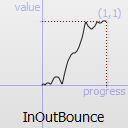Easing curve for a bounce (exponentially decaying parabolic bounce) function easing in/out: acceleration until halfway, then deceleration.

Constant

Description

QEasingCurve.OutInBounce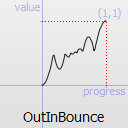Easing curve for a bounce (exponentially decaying parabolic bounce) function easing out/in: deceleration until halfway, then acceleration.

Constant

Description

QEasingCurve.BezierSpline

Allows defining a custom easing curve using a cubic bezier spline

QEasingCurve.TCBSpline

Allows defining a custom easing curve using a TCB spline

QEasingCurve.Custom

This is returned if the user specified a custom curve type with `setCustomType()` . Note that you cannot call `setType()` with this value, but `type()` can return it.

Parameters:

Adds a segment of a cubic bezier spline to define a custom easing curve. It is only applicable if `type()` is `BezierSpline` . Note that the spline implicitly starts at (0.0, 0.0) and has to end at (1.0, 1.0) to be a valid easing curve. `c1` and `c2` are the control points used for drawing the curve. `endPoint` is the endpoint of the curve.

Parameters:

Adds a segment of a TCB bezier spline to define a custom easing curve. It is only applicable if `type()` is `TCBSpline` . The spline has to start explicitly at (0.0, 0.0) and has to end at (1.0, 1.0) to be a valid easing curve. The tension `t` changes the length of the tangent vector. The continuity `c` changes the sharpness in change between the tangents. The bias `b` changes the direction of the tangent vector. `nextPoint` is the sample position. All three parameters are valid between -1 and 1 and define the tangent of the control point. If all three parameters are 0 the resulting spline is a Catmull-Rom spline. The begin and endpoint always have a bias of -1 and 1, since the outer tangent is not defined.

PySide6.QtCore.QEasingCurve.amplitude()#
Return type:

float

Returns the amplitude. This is not applicable for all curve types. It is only applicable for bounce and elastic curves (curves of `type()` `InBounce` , `OutBounce` , `InOutBounce` , `OutInBounce` , `InElastic` , `OutElastic` , `InOutElastic` or `OutInElastic` ).

PySide6.QtCore.QEasingCurve.customType()#
Return type:

object

PySide6.QtCore.QEasingCurve.__ne__(other)#
Parameters:
Return type:

bool

Compare this easing curve with `other` and returns `true` if they are not equal. It will also compare the properties of a curve.

`operator==()`

PySide6.QtCore.QEasingCurve.__eq__(other)#
Parameters:
Return type:

bool

Compare this easing curve with `other` and returns `true` if they are equal. It will also compare the properties of a curve.

PySide6.QtCore.QEasingCurve.overshoot()#
Return type:

float

Returns the overshoot. This is not applicable for all curve types. It is only applicable if `type()` is `InBack` , `OutBack` , `InOutBack` or `OutInBack` .

PySide6.QtCore.QEasingCurve.period()#
Return type:

float

Returns the period. This is not applicable for all curve types. It is only applicable if `type()` is `InElastic` , `OutElastic` , `InOutElastic` or `OutInElastic` .

PySide6.QtCore.QEasingCurve.setAmplitude(amplitude)#
Parameters:

amplitude – float

Sets the amplitude to `amplitude`.

This will set the amplitude of the bounce or the amplitude of the elastic “spring” effect. The higher the number, the higher the amplitude.

PySide6.QtCore.QEasingCurve.setCustomType(arg__1)#
Parameters:

arg__1 – object

PySide6.QtCore.QEasingCurve.setOvershoot(overshoot)#
Parameters:

overshoot – float

Sets the overshoot to `overshoot`.

0 produces no overshoot, and the default value of 1.70158 produces an overshoot of 10 percent.

PySide6.QtCore.QEasingCurve.setPeriod(period)#
Parameters:

period – float

Sets the period to `period`. Setting a small period value will give a high frequency of the curve. A large period will give it a small frequency.

PySide6.QtCore.QEasingCurve.setType(type)#
Parameters:

type`Type`

Sets the type of the easing curve to `type`.

PySide6.QtCore.QEasingCurve.swap(other)#
Parameters:

Swaps curve `other` with this curve. This operation is very fast and never fails.

PySide6.QtCore.QEasingCurve.toCubicSpline()#
Return type:

Returns the cubicBezierSpline that defines a custom easing curve. If the easing curve does not have a custom bezier easing curve the list is empty.

PySide6.QtCore.QEasingCurve.type()#
Return type:

`Type`

Returns the type of the easing curve.

PySide6.QtCore.QEasingCurve.valueForProgress(progress)#
Parameters:

progress – float

Return type:

float

Return the effective progress for the easing curve at `progress`. Whereas `progress` must be between 0 and 1, the returned effective progress can be outside those bounds. For example, `InBack` will return negative values in the beginning of the function.